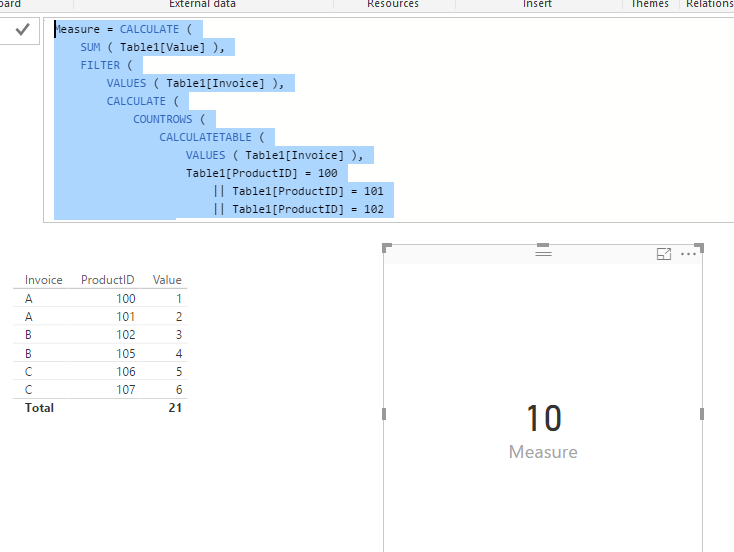cancel
Showing results for
Did you mean:Frequent Visitor

## Calculating total value of invoices where certain Product ID's exist

Hello!

I have a question that I haven’t been able to figure out nor find an answer to on the internet… so I thought I could ask here and someone may have an answer for me!

I need to calculate the total value of all invoices that contain certain Product ID’s.

Note: Just in case someone jumps the gun, I know how to use the calculate function to find the total value of certain product ID’s. Find below how I can do this.

CALCULATE([Sales]

,FILTER(

ALL('Product'[Product ID])

,'Product'[Product ID]="100"

||'Product'[Product ID]="101"

||'Product'[Product ID]="102")

)

However this is not what I am after unfortunately. I need the total value of invoices where these Product ID's exist.

If anyone has any idea it would be much appreciated!

1 ACCEPTED SOLUTIONMicrosoft

@Evan4

In this scenario, you need to use a CALCULATETABLE() to filter the corresponding Invoice first. Then use it to filter source table for your calculation. Please refer to my sample below:

```Measure = CALCULATE (
SUM ( Table1[Value] ),
FILTER (
VALUES ( Table1[Invoice] ),
CALCULATE (
COUNTROWS (
CALCULATETABLE (
VALUES ( Table1[Invoice] ),
Table1[ProductID] = 100
|| Table1[ProductID] = 101
|| Table1[ProductID] = 102
)
)
)
)
)```Regards,Microsoft

@Evan4

In this scenario, you need to use a CALCULATETABLE() to filter the corresponding Invoice first. Then use it to filter source table for your calculation. Please refer to my sample below:

```Measure = CALCULATE (
SUM ( Table1[Value] ),
FILTER (
VALUES ( Table1[Invoice] ),
CALCULATE (
COUNTROWS (
CALCULATETABLE (
VALUES ( Table1[Invoice] ),
Table1[ProductID] = 100
|| Table1[ProductID] = 101
|| Table1[ProductID] = 102
)
)
)
)
)```Regards,